# Alternative Sample Sizes for Verification Dose Experiments and Dose Audits

Dr. Wayne A. Taylor
Joyce M. Hansen

ABSTRACT

ISO 11137 (1995), “Sterilization of Health Care Products – Requirements for Validation and Routine Control – Radiation Sterilization”, provides sampling plans for performing initial verification dose experiments and quarterly dose audits.  Alternative sampling plans are presented which provide equivalent protection.  These sampling plans can significantly reduce the cost of testing. These alternative sampling plans have been included in a draft ISO Technical Report (type 2)

This paper examines the rationale behind the proposed alternative sampling plans.  The protection provided by the current verification and audit sampling plans is first examined.  Then methods for identifying equivalent plans are highlighted.  Finally, methods for comparing the cost associated with the different plans are provided.  This paper includes additional guidance for selecting between the original and alternative sampling plans not included in the technical report.

KEYWORDS

Verification Dose Experiments, Dose Audits, Radiation Sterilization, Acceptance Sampling, OC Curves, ASN Curves, Double Sampling Plans, Quick Switching Systems.

INTRODUCTION

The minimum sterilization dose depends on the product bioburden, i.e., the number and type of microorganisms found on the product.  The bioburden is influenced by raw materials along with manufacturing personnel, environment and procedures.  It may change over time.  Therefore, the resistance of these microorganisms to radiation must be monitored on an ongoing basis.  ISO 11137 (1995), “Sterilization of Health Care Products – Requirements for Validation and Routine Control – Radiation Sterilization”, provides three methods for determining the sterilization dose.  This paper addresses the procedures used by Methods 1 and 2 to monitor bioburden resistance.

To evaluate the resistance of the microorganisms, a specified number of product units are irradiated at a dose that is less than the normal sterilization dose.  This dose, called the verification dose, is calculated to give a Sterility Assurance Level (SAL) of 10-2.  The SAL is the probability that a unit of product contains one or more viable microorganisms.  Units containing viable microorganisms will exhibit growth following a test of sterility.  This growth is referred to as a positive. At a SAL of 10-2, one would expect on average that 1% of the units will test positive.  A larger than expected number of units testing positive indicates that the resistance may be greater than assumed.

The bioburden resistance is initially tested using the verification dose experiment.  Then, dose audits are performed quarterly to ensure the bioburden resistance has not increased.  Both procedures are attribute acceptance sampling plans.  This article uses the theory of acceptance sampling to evaluate the protection provided by these two procedures and then to identify alternate sampling plans that provide equivalent protection but may do so at a lower cost.  The plans so selected can reduce the number of units tested by as much as 50%. This article expands on Phillips, Taylor, Sargent, & Hansen (1996).

METHOD 1 VERIFICATION DOSE EXPERIMENT

The Method 1 verification dose experiment requires that 100 product units be irradiated at the verification dose and a test of sterility performed on each product unit.  The results are acceptable if no more than two units test positive.  This procedure is an attribute single sampling plan with sample size n=100 and accept number a=2 (Taylor, 1992).  What protection does this sampling plan provide?  To understand its protection, one must understand how it behaves. First, assume the bioburden resistance is such that 1% of the units test positive. Figure 1 shows the results of the repeated application of this sampling plan.  92% of the time the verification dose experiment is passed.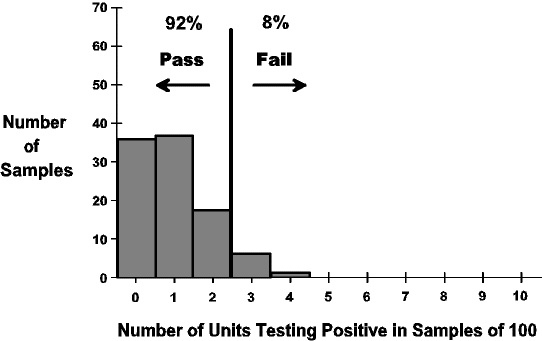Figure 1.   Behavior verification dose experiment for a 1% positive rate

Now assume the bioburden resistance increases to cause a 3% positive rate.  Figure 2 shows the expected behavior.  Now only 42% pass the verification dose experiment.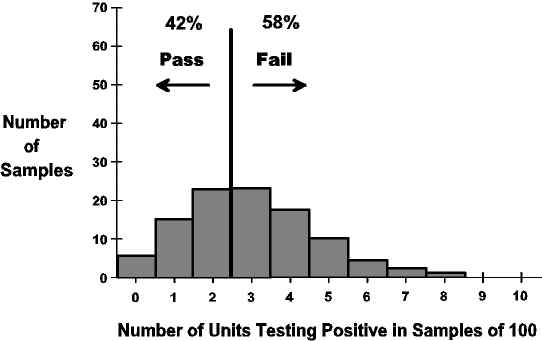Figure 2. Behavior verification dose experiment for a 3% positive rate

Finally assume the bioburden resistance increase even further to a 6% positive rate.  Figure 3 shows the expected behavior.  In this case, only 6% pass the verification dose experiment.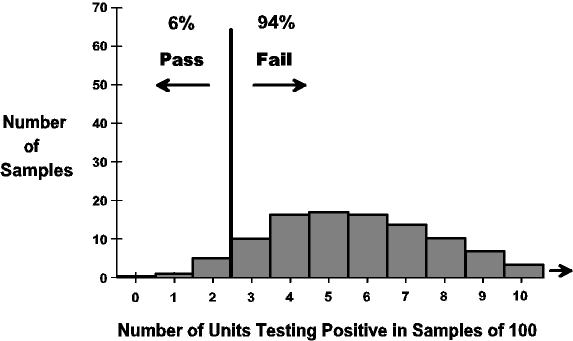Figure 3. Behavior verification dose experiment for a 6% positive rate

Summarizing the behavior of the verification dose experiment, a 1% positive rate routinely passes, a 6% positive rate routinely fails, while a 3% positive rate sometimes passes and sometimes fails.  The behavior of a sampling plan is generally displayed in the form of an Operating Characteristic Curve (OC Curve).  The OC curve of the verification dose experiment is shown in Figure 4.  The bottom axis gives different positive rates.  The left axis gives the corresponding probability of passing.  The three cases covered in Figures 1-3 are highlighted.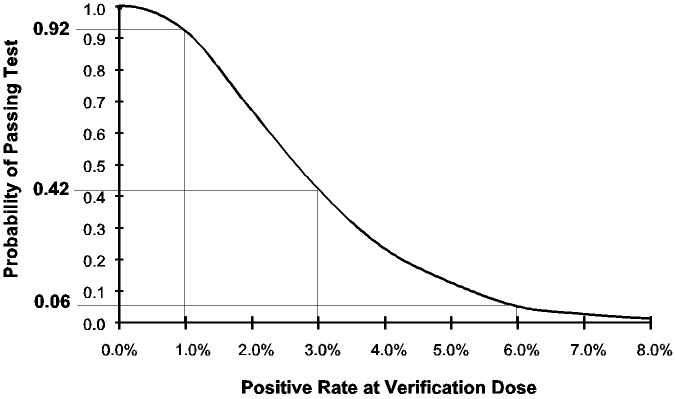Figure 4. OC curve of Method 1 verification dose experiment

Rather than drawing the OC of each sampling plan, the protection provided by a sampling plan is often summarized using two points on the OC curve called the AQL and LTPD.  The AQL (Acceptable Quality Level) is that positive rate that has a 95% chance of passing.  For the verification dose experiment, the AQL is 0.823% (See Figure 5).  The AQL represent a positive rate routinely passed by the sampling plan.  The LTPD (Lot Tolerance Percent Defective) is that positive rate that has a 10% chance of passing (90% chance of failing).  For the verification dose experiment, the LTPD is 5.23%.  The LTPD represents a positive rate routinely failed by the sampling plan.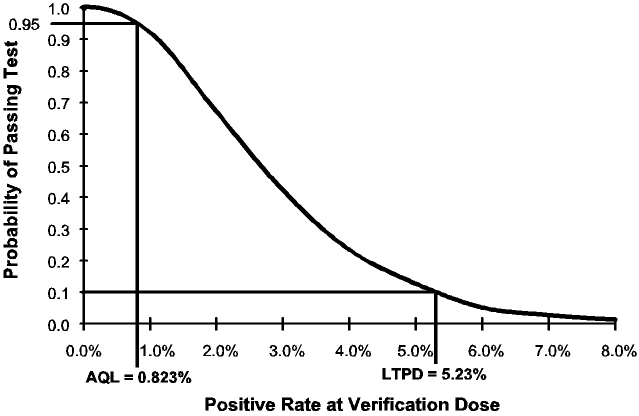Figure 5. AQL and LTPD of Method 1 verification dose experiment

Together the AQL and LTPD summarize the protection of the verification dose experiment.  It routinely passes when the positive rate is 0.823% (AQL) or better.  It routinely fails when the positive rate is 5.23% (LTPD) or higher.  It sometimes passes and sometimes fails when the positive rate is between the AQL and LTPD.  Ideally, we would like the verification dose experiment to always pass if the positive rate is 1% or less and to always fail if the positive rate is above 1%.  However, since the decision must be based on a sample, there is always a risk of making an incorrect decision.  As a result, there is always a chance of passing when the positive rate is above 1%.  There is also a chance of failing when the positive rate is below 1%.  Together, the AQL, LTPD and OC curves describe these risks.

Other sampling plans exist which have similar AQLs and LTPDs. One such plan is the attribute double sampling plan:

Alternative Sampling Plan – Verification Dose Experiment

First sample size: n1 = 52
First accept number: a1 = 0
First reject number: r1 = 3
Second sample size: n2 = 52
Second accept number: a2 = 2

This plan works as follows.  Initially, 52 (n1) units are sterilized at the verification dose and the number of positive sterility tests tallied.  If 0 (a1) positives are found, the verification dose experiment passes.  If 3 (r1) or more positives are found, the verification dose experiment is not passed.  In the event that 1 or 2 positives are found, 52 (n2) more units are sterilized at the verification dose and sterility tested.  If the total number of positives in the combined sample of 102 units is 2 (a2) or less, the verification dose experiment passes.  If the total number of positives in the combined sample of 102 units is 3 or greater, the verification dose experiment is not passed.  The OC curve, AQL and LTPD of this alternative double sampling plan is shown in Figure 6.  This sampling plan was obtained using the software accompanying Taylor (1992).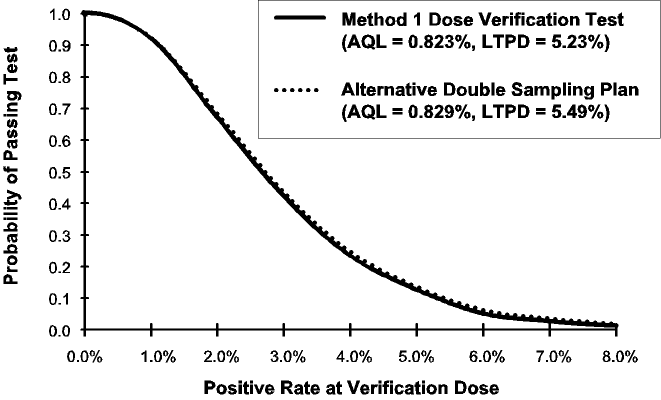Figure 6: OC curves of Method 1 verification dose experiment and alternative double sampling plan

If two sampling plans have similar AQLs and LTPDs, they will have similar OC curves.  They will provide the same protection against an increase in bioburden resistance.  They will also falsely fail reduced bioburden resistance at the same rate.  The protection and risks are similar.  As a result, the two sampling plans are substantially equivalent procedures.

Figure 7 shows the Average Sample Number (ASN) curves of these two sampling plans.  The bottom axis gives different positive rates. The left axis gives the corresponding average number of units tested.  The current verification dose experiment is a single sampling plan that always requires 100 units.  However, the number of units required by the alternative double sampling plan varies. If the positive rate is near zero, the verification dose experiment generally passes after taking the initial 52 samples.  As the positive rate starts to increase, the second sample will be required more often as the ASN curve starts to increase.  As the positive rate increases further, the verification dose experiment will fail more frequently on the first sample.  As a result, the ASN curves starts dropping again till it again approaches 52.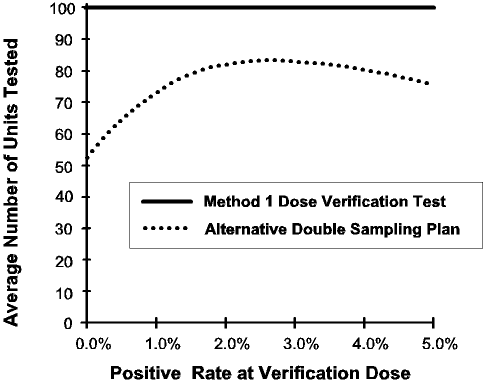Figure 7: ASN curves of Method 1 verification dose experiment and alternative double sampling plan

When comparing ASN curves, you should concentrate on that region corresponding to the average positive rate that you have observed over time.  In Hansen (1993), it is estimated that the industry average positive rate is around 0.5%.  At 0.5%, the double sampling plan averages 63.8 units tested compared to 100 for the current procedure.  This is a 46% reduction in the number of units tested.

The number of units tested is not the only consideration.  The double sampling is a more complex procedure that sometimes requires an additional irradiation processing of samples.  Figure 8 shows the probability of a second sample for the two procedures.  The current verification dose experiment is a single sampling plan that never requires a second sample.  Therefore the probability is always 0%.  However, the probability of a second sample for the alternative double sampling plan varies depending on the positive rate.  At a positive rate of 0.5%, the probability of a second sample is 0.2272.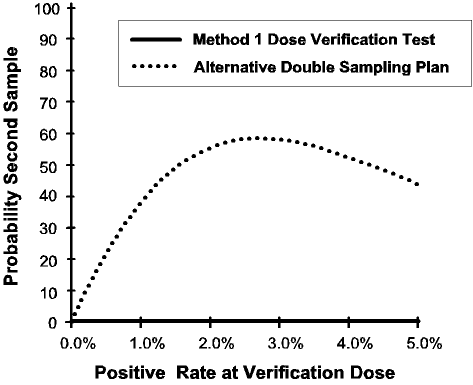Figure 8: Probability of second sample of Method 1 verification dose experiment and alternative double sampling plan

So which plan is best?  This depends on the cost associated with testing an individual unit (cunit) and the cost of going to the second stage (cstage).  When the cost of testing the individual units is the predominant cost, the alternative double sampling plan is preferred.  When the cost of going to the second stage is the predominant cost, the current single sampling plan is preferred.  Neither plan is best for all situations.

The total inspection costs (ctotal) associated with the two plans are:

Single sampling plan: ctotal = 100 cunit

Double sampling plan: ctotal = ASN(p) cunit +P2 (p) cstage

where ASN(p) is the average sample number and P2(p) is the probability of the second stage at a positive rate of p.  When the positive rate is 0.5%, ASN(0.5%) = 63.8 and P2(0.5%) is 0.2272.  In this case, the best plan is:

Verification Dose Experiment – Choosing the Best Sampling Plan for a 0.5% Positive Rate

The Method 1 single sampling plan is best when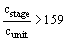The alternative double sampling plan is best when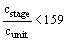For a positive rate of 0.5%, Figure 9 compares the total cost of the two sampling plans dependent on the ratio of the two inspection costs.  The point of intersection of these two curves is 159.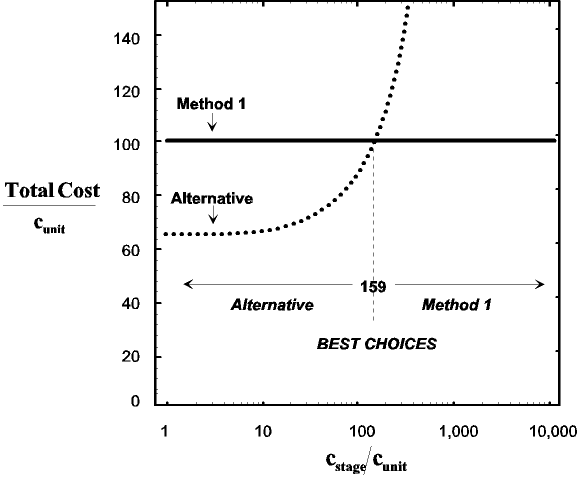Figure 9: Cost comparison of Method 1 verification dose experiment
and alternative double sampling plan for a positive rate of 0.5%

DOSE AUDIT

Dose audits are required for both Methods 1 and 2.  The dose audit requires that 100 product units be irradiated at a 10-2 SAL dose and a test of sterility performed on each product unit.  The results are acceptable if 2 or fewer units test positive.  If 3 or 4 positives are found, a retest is allowed.  Essentially this is a double sampling plan.

Dose Audit as Double Sampling Plan

First sample size: n1 = 100
First accept number: a1 = 2
First reject number: r1 = 5
Second sample size: n2 = 100
Second accept number: a2 = 2

This is a nonstandard double sampling plan in that a2* applies only to the number of positives in the second sample rather than the normal procedure where it applies the cumulative number of positives in both samples.  The OC curve, AQL and LTPD of the dose audit are given in Figure 10.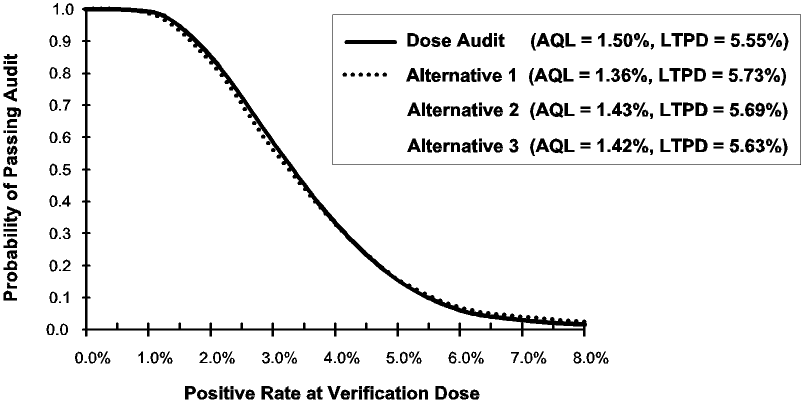Figure 10: OC curves of dose audit and alternative 1 double sampling plans

Three alternative sampling plans are shown below.  One is a single sampling plan and the other two are standard double sampling plans.

Dose Audit – Alternative 1

First sample size: n1 = 50
First accept number: a1 = 0
First reject number: r1 = 4
Second sample size: n2 = 100
Second accept number: a2 = 4

Dose Audit – Alternative 2

First sample size: n1 = 70
First accept number: a1 = 1
First reject number: r1 = 6
Second sample size: n2 = 130
Second accept number: a2 = 5

Dose Audit – Alternative 3

Sample size: n = 140
Accept number: a = 4

The OC curves, AQLs and LTPDs of the three alternative plans are also shown in Figure 10.  These three procedures are substantially equivalent to the dose audit procedure including retest.  While the protection is the same, the costs are not. Figure 11 shows the ASN curves of these procedures and Figure 12 shows the probabilities of second samples . Alternative 1 can reduce the number of units tested by 29% (from 101.4 to 72.2).  On the other hand, alternative 3 can eliminate the need for a second sample.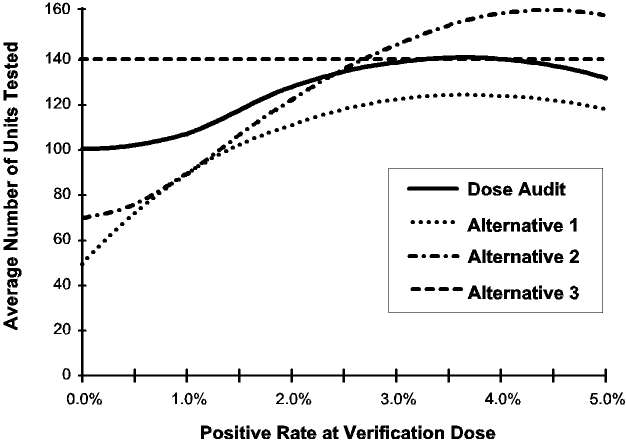Figure 11: ASN curves of dose audit and alternative sampling plans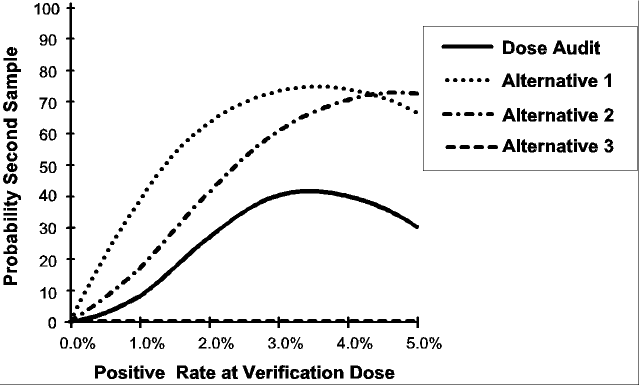Figure 12: Probability of second sample of dose audit and alternative sampling plans

Figure 13 compares the total cost of the four procedures when the positive rate is 0.5%.  Criteria for selecting the best plan are given below.  Modifications and corrections to the criteria given in Phillips (1996) were suggested by Kyprianou (1996).

Dose Audit – Choosing the Best Sampling Plan for a 0.5% Positive Rate

The alternative 1 double sampling plan is best when: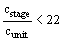The alternative 2 double sampling plan is best when: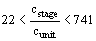The Method 1 retest procedure is best when: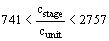The alternative 3 single sampling plan is best when: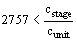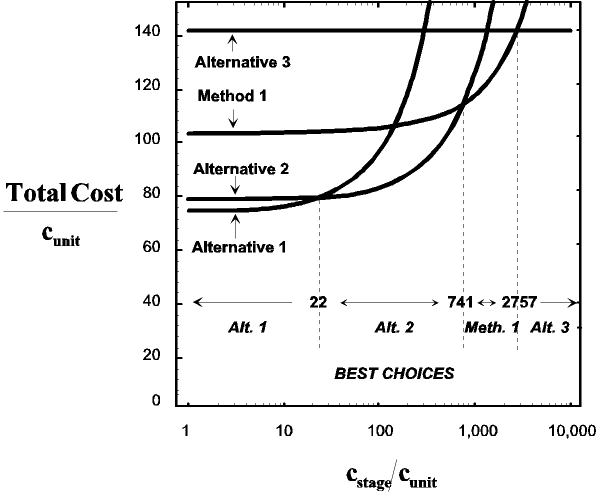Figure 13: Cost comparison of dose audit and alternative sampling plans for a positive rate of 0.5%

QUICK SWITCHING SYSTEMS

The Method 1 verification dose experiment combined with the dose audit is a quick switching system (QSS).  QSSs are investigated in Taylor (1992, 1996).  A QSS consists of two sampling plans along with a set of rules for switching between them.  The first plan, called the reduced plan, is intended for use when the process is running well.  The dose audit sampling plan serves the role of the reduced plan.  The second plan, called the tightened plan, is intended for use when a problem is encountered.  One always starts a QSS with the tightened plan. The verification dose experiment sampling plan serves the role of the tightened plan.  A QSS has a set of rules for switching between the two plans.  Figure 14 shows the Method 1 verification dose experiment and dose audit represented as a QSS.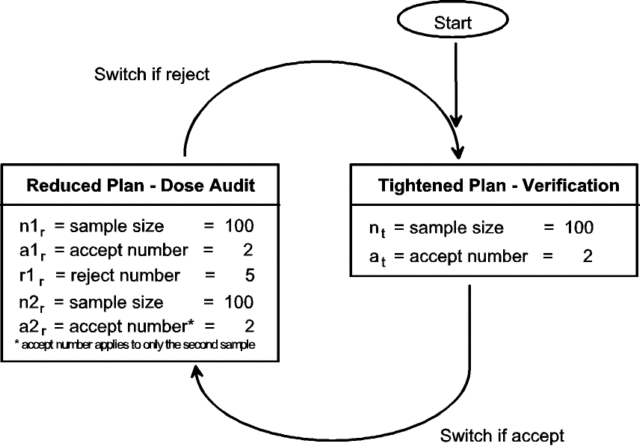Figure 14: Method 1 as a quick switching system

While Method 1 is a QSS, it differs from most QSSs in that the reduced inspection actually requires more units to be tested than the tightened plan.  This is a result of the fact that it has a steeper OC curve.  Generally, the tightened inspection requires the greater number of units in order to provide superior protection.  It is obviously preferable to detect a sterilization dose problem before it is used, rather than after several months.

When designing a QSS, one must protect against the bioburden resistance initially exceeding that of the theoretical bioburden resistance distribution.  One must also protect against future increases in resistance.  If both situations are equally likely, a more traditional QSS with tighter initial inspection makes sense.  Using this approach, an alternative QSS was selected using the software accompanying Taylor (1992).  This QSS is shown in Figure 15.  It was selected to offer: (1) slightly increased protection against an initially high level of bioburden resistance, (2) slightly decreased protection following a sudden increase in the bioburden resistance, and (3) equivalent protection against more gradual changes in the bioburden resistance.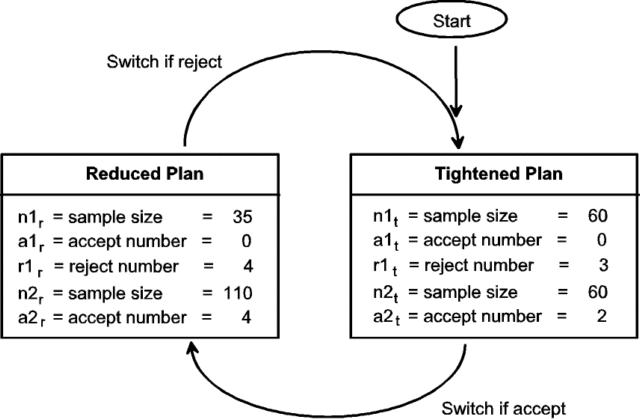Figure 15: Alternative quick switching system

The scheme or stationary OC curves for the two QSSs are shown in Figure 16.  The stationary OC curve of a QSS describes its protection during periods where the positive rate is constant or changing gradually.  Because their OC curves are nearly the same, both QSSs offer equivalent protection under this scenario.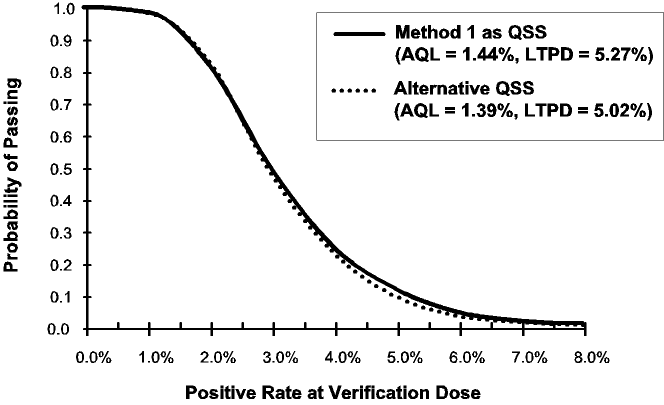Figure 16: Stationary OC curves

Of special importance is the protection provided during start-up.  Figure 17 shows the OC curves of the different tightened plans used at startup.  Since its OC curve is lower, the alternative QSS offers slightly better protection.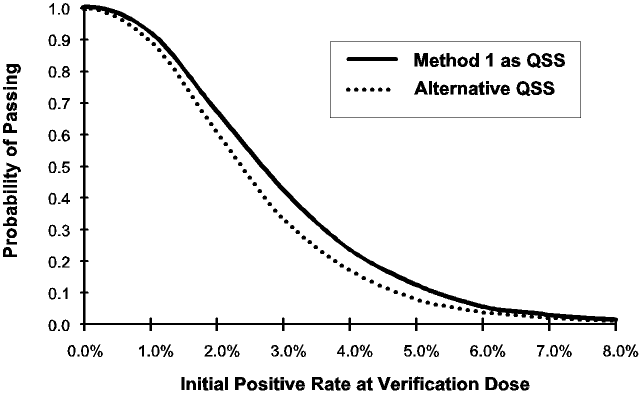Figure 17: Start-up protection

Finally, one must be concerned with the protection provided following a sudden increase in the bioburden resistance.  Suppose the QSS is in its reduced state when the resistance suddenly increases.  Figure 18 shows the probabilities of passing the first dose audit following such an increase.  The alternative QSS offers slightly decreased protection.  However, this decreased protection only occurs following a jump in resistance between one quarterly audit and the next.  When the resistance trends upward over time, the stationary OC curve better describes the protection, in which case both plans offer equivalent protection.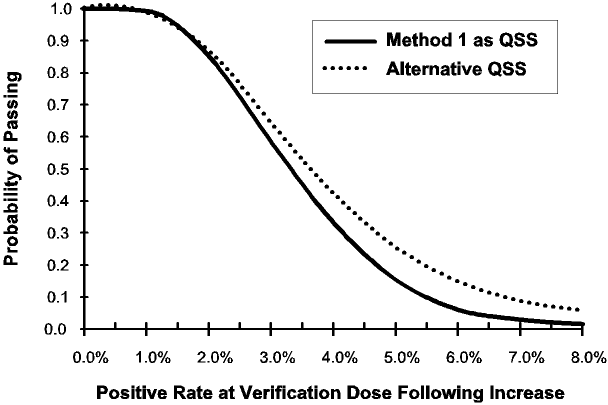Figure 18: Protection following increase in positive rate

Figures 16 to 18 show that the alternative QSS offers increased protection under certain scenarios while offering decreased protection under others. In no case is the difference large. Taken all together, the alternative QSS provides equivalent protection. While the alternative QSSs offers equivalent protection, it differs greatly in terms of the number of units tested. Figure 19 shows the ASN curves of these two QSSs. At a 0.5% positive rate, the alternative QSS decreases the number of units tested by 55%.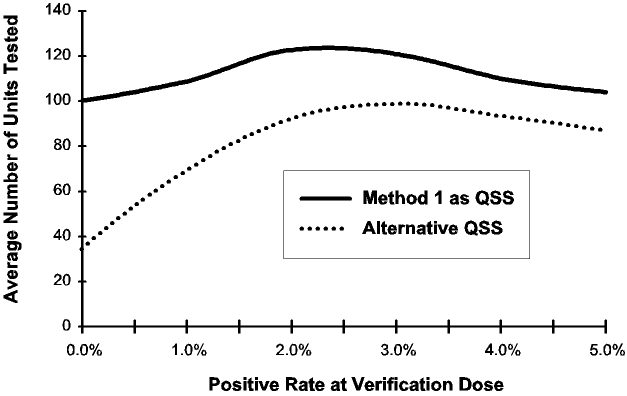Figure 19: ASN curves of method 1 and alternative quick switching system

CONCLUSION

The alternative sampling plans presented in this article provide equivalent protection to the current procedures for the verification dose experiment and dose audit.  While they offer the same protection, the procedures result in different testing costs.  These alternative sampling plans may significantly reduce the costs of performing this testing.  No one plan is best for all situations.  The best sampling plan depends on the per unit cost of testing and the cost of going to a second sample.  Criteria are provided for deciding which sampling plan is best suited for a particular application.

REFERENCES

Hansen, J. (1993). “AAMI Dose Setting: Ten Years Experience in Sterilization of Medical Products.” Proceedings of Kilmer Conference, Brussels, Belgium.

ISO 11137 (1995), “Sterilization of Health Care Products – Requirements for Validation and Routine Control – Radiation Sterilization”, International Organization for Standardization, Switzerland.

Kyprianou, E. (1996), A review of the article “Reducing sample sizes of AAMI Gamma Radiation sterilization verification experiments and dose audits”, private correspondence.

Phillips, G.W.; Taylor, W. A.; Sargent, H. E. and Hansen, J. M. (1996), “Reducing Sample Sizes of AAMI Gamma Radiation Sterilization Verification Experiments and Dose Audits,” Quality Engineering, Volume 8, Number 3, pp. 489-496,.

Taylor, Wayne A. (1992). Guide to Acceptance Sampling, Taylor Enterprises, Inc., Libertyville, Illinois.

Taylor, Wayne A. (1996). “Quick Switching Systems,” Journal of Quality Technology, Vol. 28, No. 3, pp. 460-472.

Presented at 10th International Meeting on Radiation Processing, Anaheim, California, 1997

To appear in Radiation Physics and Chemistry, Elsevier Science Ltd., Exeter, UK